带阻滤波器_带阻滤波器传递函数 - CSDN

• ## 理想带阻滤波器

千次阅读 2016-07-01 18:44:58
理想带阻滤波器是频域滤波的一种，主要是抑制距离频域中心D0、一个圆环区域的频域成分，因此可以使用理想带阻滤波器来消除频率分布在圆环上的周期噪声。 公式如下   产生的3D理想带阻滤波器的图形如下    ...

理想带阻滤波器是频域滤波的一种，主要是抑制距离频域中心D0、一个圆环区域的频域成分，因此可以使用理想带阻滤波器来消除频率分布在圆环上的周期噪声。
公式如下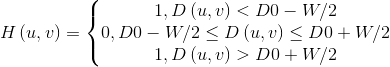产生的3D理想带阻滤波器的图形如下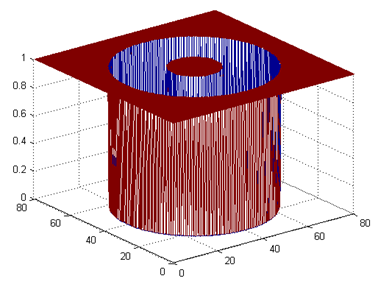现在看一下对一个图像加点白噪声，然后滤波一下，下面是原图和滤波后的图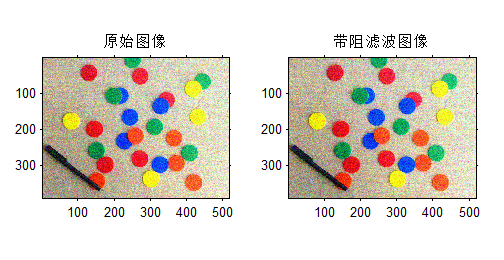展开全文理想带阻滤波器 matlab
• 下两个滤波器都是切比雪夫I型数字滤波器，不是巴特沃尔滤波器，请使用者注意！1.低通滤波器使用说明：将下列代码幅值然后以m文件保存，文件名要与函数名相同，这里函数名：lowp。function y=lowp(x,f1,f3,rp,rs,Fs) ...
本文为转载内容，原文地址为点击打开链接。下两个滤波器都是切比雪夫I型数字滤波器，不是巴特沃尔滤波器，请使用者注意！1.低通滤波器使用说明：将下列代码幅值然后以m文件保存，文件名要与函数名相同，这里函数名：lowp。function y=lowp(x,f1,f3,rp,rs,Fs)
%低通滤波
%使用注意事项：通带或阻带的截止频率的选取范围是不能超过采样率的一半
%即，f1,f3的值都要小于 Fs/2
%x:需要带通滤波的序列
% f 1：通带截止频率
% f 3：阻带截止频率
%rp：边带区衰减DB数设置
%rs：截止区衰减DB数设置
%FS：序列x的采样频率
% rp=0.1;rs=30;%通带边衰减DB值和阻带边衰减DB值
% Fs=2000;%采样率
%
wp=2*pi*f1/Fs;
ws=2*pi*f3/Fs;
% 设计切比雪夫滤波器；
[n,wn]=cheb1ord(wp/pi,ws/pi,rp,rs);
[bz1,az1]=cheby1(n,rp,wp/pi);
%查看设计滤波器的曲线
[h,w]=freqz(bz1,az1,256,Fs);
h=20*log10(abs(h));
figure;plot(w,h);title('所设计滤波器的通带曲线');grid on;
%
y=filter(bz1,az1,x);%对序列x滤波后得到的序列y
end--------------------------------------低通滤波器使用例子的代码fs=2000;
t=(1:fs)/fs;
ff1=100;
ff2=400;
x=sin(2*pi*ff1*t)+sin(2*pi*ff2*t);
figure;
subplot(211);plot(t,x);
subplot(212);hua_fft(x,fs,1);
%低通测试
% y=filter(bz1,az1,x);
y=lowp(x,300,350,0.1,20,fs);
figure;
subplot(211);plot(t,y);
subplot(212);hua_fft(y,fs,1);%hua_fft()函数是画频谱图的函数，代码在下面给出，要保存为m文件调用%这段例子还调用了我自己写的专门画频谱图的函数，也给出，不然得不出我的结果;%画信号的幅频谱和功率谱
%频谱使用matlab例子表示
function hua_fft(y,fs,style,varargin)
%当style=1,画幅值谱；当style=2,画功率谱;当style=其他的，那么花幅值谱和功率谱
%当style=1时，还可以多输入2个可选参数
%可选输入参数是用来控制需要查看的频率段的
%第一个是需要查看的频率段起点
%第二个是需要查看的频率段的终点
%其他style不具备可选输入参数，如果输入发生位置错误
nfft=2^nextpow2(length(y));%找出大于y的个数的最大的2的指数值（自动进算最佳FFT步长nfft）
%nfft=1024;%人为设置FFT的步长nfft
y=y-mean(y);%去除直流分量
y_ft=fft(y,nfft);%对y信号进行DFT，得到频率的幅值分布
y_p=y_ft.*conj(y_ft)/nfft;%conj()函数是求y函数的共轭复数，实数的共轭复数是他本身。
y_f=fs*(0:nfft/2-1)/nfft;�T变换后对应的频率的序列
% y_p=y_ft.*conj(y_ft)/nfft;%conj()函数是求y函数的共轭复数，实数的共轭复数是他本身。
if style==1
ifnargin==3
plot(y_f,2*abs(y_ft(1:nfft/2))/length(y));%matlab的帮助里画FFT的方法
%ylabel('幅值');xlabel('频率');title('信号幅值谱');
%plot(y_f,abs(y_ft(1:nfft/2)));%论坛上画FFT的方法
else
f1=varargin{1};
fn=varargin{2};
ni=round(f1 * nfft/fs+1);
na=round(fn * nfft/fs+1);
plot(y_f(ni:na),abs(y_ft(ni:na)*2/nfft));
end

elseif style==2
plot(y_f,y_p(1:nfft/2));
%ylabel('功率谱密度');xlabel('频率');title('信号功率谱');
else
subplot(211);plot(y_f,2*abs(y_ft(1:nfft/2))/length(y));
ylabel('幅值');xlabel('频率');title('信号幅值谱');
subplot(212);plot(y_f,y_p(1:nfft/2));
ylabel('功率谱密度');xlabel('频率');title('信号功率谱');
end
end下面三幅图分别是滤波前的时频图，滤波器的滤波特性曲线图和滤波后的时频图，通过图可以看出成功留下了100Hz的低频成分而把不要的高频成分去除了。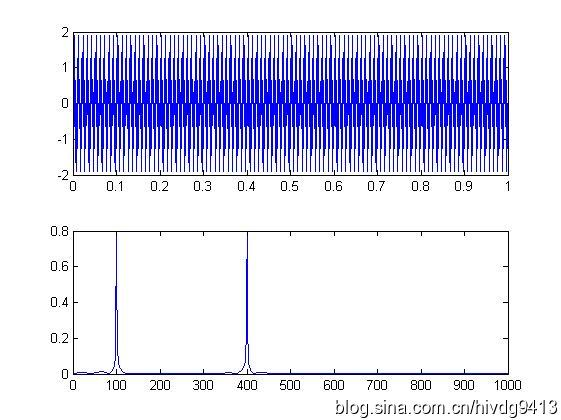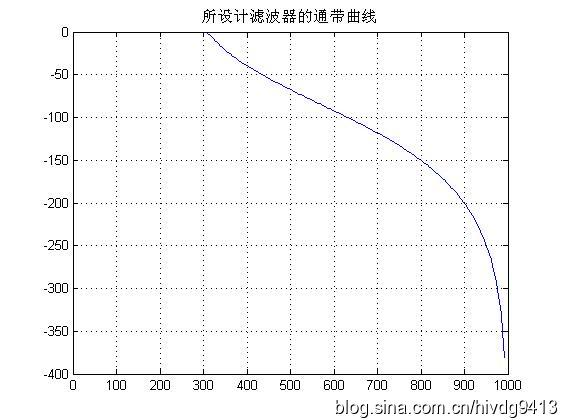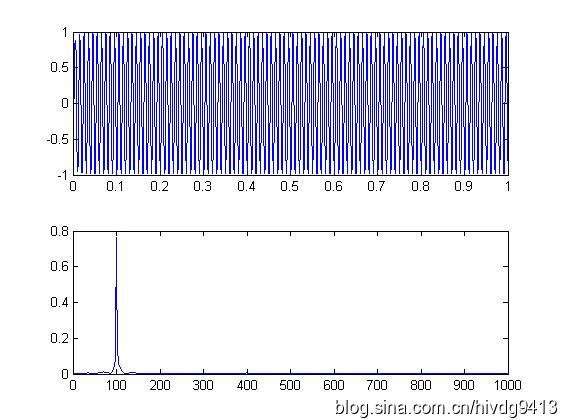2.高通滤波器function y=highp(x,f1,f3,rp,rs,Fs)
%高通滤波
%使用注意事项：通带或阻带的截止频率的选取范围是不能超过采样率的一半
%即，f1,f3的值都要小于 Fs/2
%x:需要带通滤波的序列
% f 1：通带截止频率
% f 2：阻带截止频率
%rp：边带区衰减DB数设置
%rs：截止区衰减DB数设置
%FS：序列x的采样频率
% rp=0.1;rs=30;%通带边衰减DB值和阻带边衰减DB值
% Fs=2000;%采样率
%
wp=2*pi*f1/Fs;
ws=2*pi*f3/Fs;
% 设计切比雪夫滤波器；
[n,wn]=cheb1ord(wp/pi,ws/pi,rp,rs);
[bz1,az1]=cheby1(n,rp,wp/pi,'high');

%查看设计滤波器的曲线
[h,w]=freqz(bz1,az1,256,Fs);
h=20*log10(abs(h));
figure;plot(w,h);title('所设计滤波器的通带曲线');grid on;
y=filter(bz1,az1,x);
end下面是高通滤波器的例子fs=2000;
t=(1:fs)/fs;
ff1=100;
ff2=400;
x=sin(2*pi*ff1*t)+sin(2*pi*ff2*t);
figure;
subplot(211);plot(t,x);
subplot(212);hua_fft(x,fs,1);

%------高通测试
z=highp(x,350,300,0.1,20,fs);
figure;
subplot(211);plot(t,z);
subplot(212);hua_fft(z,fs,1);下面三幅图分别是滤波前的时频图，滤波器的滤波特性曲线图和滤波后的时频图，通过图可以看出成功留下了400Hz的高频成分而把不要的低频成分100Hz去除了。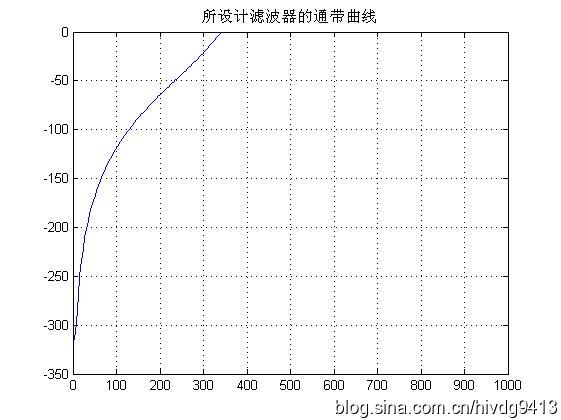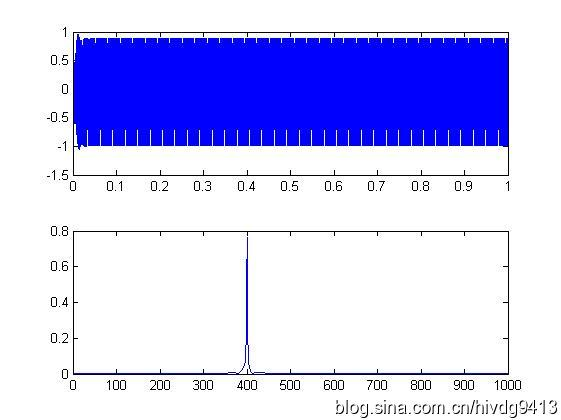3.带通滤波器function y=bandp(x,f1,f3,fsl,fsh,rp,rs,Fs)
%带通滤波
%使用注意事项：通带或阻带的截止频率与采样率的选取范围是不能超过采样率的一半
%即，f1,f3,fs1,fsh,的值小于 Fs/2
%x:需要带通滤波的序列
% f 1：通带左边界
% f 3：通带右边界
% fs1：衰减截止左边界
% fsh：衰变截止右边界
%rp：边带区衰减DB数设置
%rs：截止区衰减DB数设置
%FS：序列x的采样频率
% f1=300;f3=500;%通带截止频率上下限
% fsl=200;fsh=600;%阻带截止频率上下限
% rp=0.1;rs=30;%通带边衰减DB值和阻带边衰减DB值
% Fs=2000;%采样率
%
wp1=2*pi*f1/Fs;
wp3=2*pi*f3/Fs;
wsl=2*pi*fsl/Fs;
wsh=2*pi*fsh/Fs;
wp=[wp1 wp3];
ws=[wsl wsh];
%
% 设计切比雪夫滤波器；
[n,wn]=cheb1ord(ws/pi,wp/pi,rp,rs);
[bz1,az1]=cheby1(n,rp,wp/pi);
%查看设计滤波器的曲线
[h,w]=freqz(bz1,az1,256,Fs);
h=20*log10(abs(h));
figure;plot(w,h);title('所设计滤波器的通带曲线');grid on;
y=filter(bz1,az1,x);
end带通滤波器使用例子%--------------
%带通滤波器测试程序
fs=2000;
t=(1:fs)/fs;
ff1=100;
ff2=400;
ff3=700;
x=sin(2*pi*ff1*t)+sin(2*pi*ff2*t)+sin(2*pi*ff3*t);
figure;
subplot(211);plot(t,x);
subplot(212);hua_fft(x,fs,1);
% y=filter(bz1,az1,x);
y=bandp(x,300,500,200,600,0.1,30,fs);
figure;
subplot(211);plot(t,y);
subplot(212);hua_fft(y,fs,1);%调用到的hua_fft()函数代码如下function hua_fft(y,fs,style,varargin)
%当style=1,画幅值谱；当style=2,画功率谱;当style=其他的，那么花幅值谱和功率谱
%当style=1时，还可以多输入2个可选参数
%可选输入参数是用来控制需要查看的频率段的
%第一个是需要查看的频率段起点
%第二个是需要查看的频率段的终点
%其他style不具备可选输入参数，如果输入发生位置错误
nfft=2^nextpow2(length(y));%找出大于y的个数的最大的2的指数值（自动进算最佳FFT步长nfft）
%nfft=1024;%人为设置FFT的步长nfft
y=y-mean(y);%去除直流分量
y_ft=fft(y,nfft);%对y信号进行DFT，得到频率的幅值分布
y_p=y_ft.*conj(y_ft)/nfft;%conj()函数是求y函数的共轭复数，实数的共轭复数是他本身。
y_f=fs*(0:nfft/2-1)/nfft;�T变换后对应的频率的序列
% y_p=y_ft.*conj(y_ft)/nfft;%conj()函数是求y函数的共轭复数，实数的共轭复数是他本身。
if style==1
ifnargin==3
plot(y_f,2*abs(y_ft(1:nfft/2))/length(y));%matlab的帮助里画FFT的方法
%ylabel('幅值');xlabel('频率');title('信号幅值谱');
%plot(y_f,abs(y_ft(1:nfft/2)));%论坛上画FFT的方法
else
f1=varargin{1};
fn=varargin{2};
ni=round(f1 * nfft/fs+1);
na=round(fn * nfft/fs+1);
plot(y_f(ni:na),abs(y_ft(ni:na)*2/nfft));
end

elseif style==2
plot(y_f,y_p(1:nfft/2));
%ylabel('功率谱密度');xlabel('频率');title('信号功率谱');
else
subplot(211);plot(y_f,2*abs(y_ft(1:nfft/2))/length(y));
ylabel('幅值');xlabel('频率');title('信号幅值谱');
subplot(212);plot(y_f,y_p(1:nfft/2));
ylabel('功率谱密度');xlabel('频率');title('信号功率谱');
end
end运行结果如下图，第一幅是滤波前测试信号的时频图，第二幅是滤波器的滤波曲线图，第三幅是经滤波后的测试信号时频图。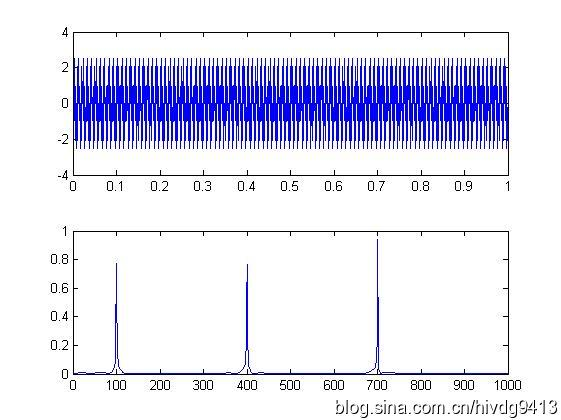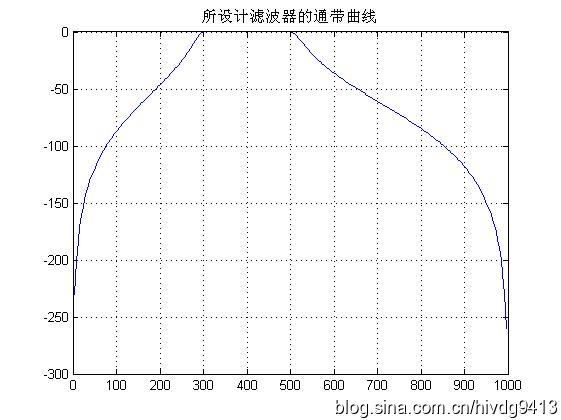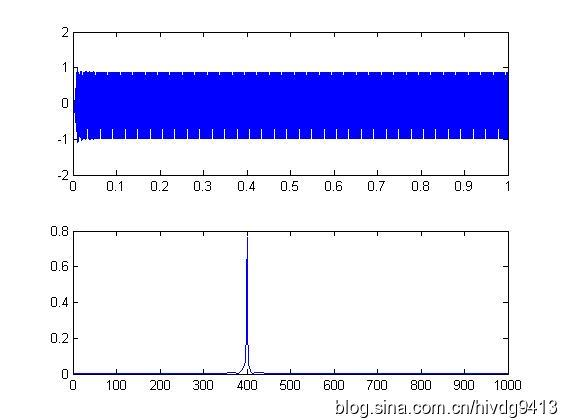4.带阻滤波器function y=bands(x,f1,f3,fsl,fsh,rp,rs,Fs)
%带阻滤波
%使用注意事项：通带或阻带的截止频率与采样率的选取范围是不能超过采样率的一半
%即，f1,f3,fs1,fsh,的值小于 Fs/2
%x:需要带通滤波的序列
% f 1：通带左边界
% f 3：通带右边界
% fs1：衰减截止左边界
% fsh：衰变截止右边界
%rp：边带区衰减DB数设置
%rs：截止区衰减DB数设置
%FS：序列x的采样频率
% f1=300;f3=500;%通带截止频率上下限
% fsl=200;fsh=600;%阻带截止频率上下限
% rp=0.1;rs=30;%通带边衰减DB值和阻带边衰减DB值
% Fs=2000;%采样率
%
wp1=2*pi*f1/Fs;
wp3=2*pi*f3/Fs;
wsl=2*pi*fsl/Fs;
wsh=2*pi*fsh/Fs;
wp=[wp1 wp3];
ws=[wsl wsh];
%
% 设计切比雪夫滤波器；
[n,wn]=cheb1ord(ws/pi,wp/pi,rp,rs);
[bz1,az1]=cheby1(n,rp,wp/pi,'stop');
%查看设计滤波器的曲线
[h,w]=freqz(bz1,az1,256,Fs);
h=20*log10(abs(h));
figure;plot(w,h);title('所设计滤波器的通带曲线');grid on;
y=filter(bz1,az1,x);
end使用例子%带阻滤波器测试
fs=1000;
t=(1:fs)/fs;
y=sin(2*pi*100*t)+sin(2*pi*150*t)+sin(2*pi*200*t);
figure;hua_fft(y,fs,1);
z=bands(y,110,190,140,160,0.1,30,fs);
figure;hua_fft(z,fs,1);运行结果如下图，第一幅是滤波前测试信号的频谱图，第二幅是滤波器的滤波曲线图，第三幅是经滤波后的测试信号频谱图。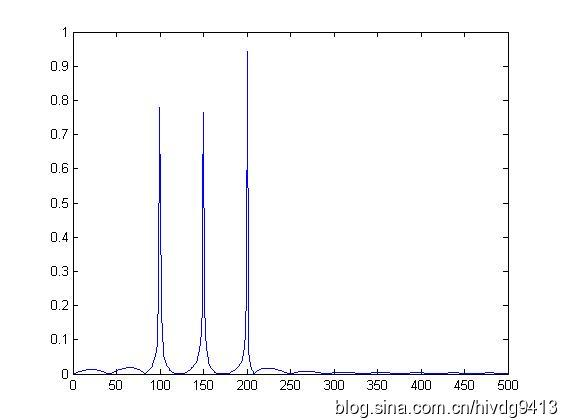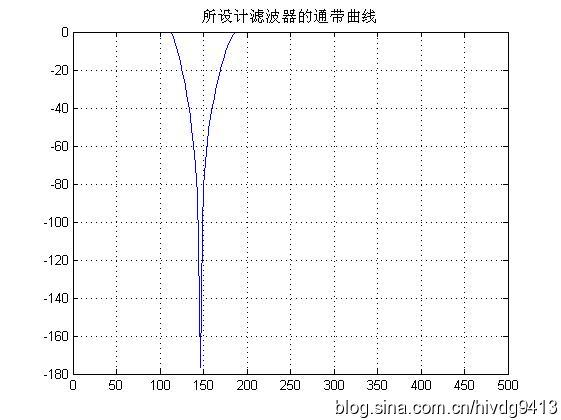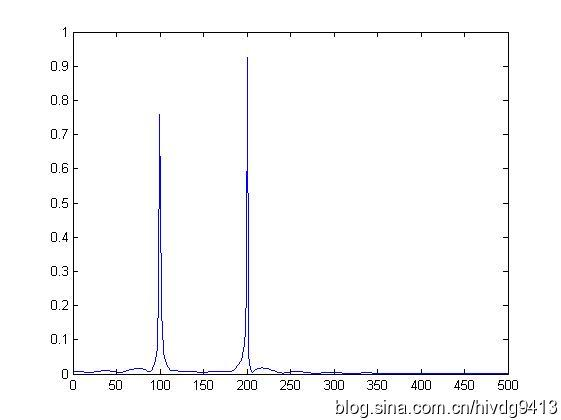展开全文低通滤波器 高通滤波器 带通滤波器 带阻滤波器 matlab
• 这个文档涉及了带通和带阻滤波器实现过程中用到的基础知识，实现公式，其中有几个实例，对于初学者能很快上手
• 先给出一张图像，来说明带阻滤波器和带通滤波器的关系。   由上图可以看出这张图片的中低成份占的比较多，而高频成份比较少。因为白色的像素都集中在中点和离中心的附近。对于频谱图，由白色代表某一频率点有响应...
先给出一张图像，来说明带阻滤波器和带通滤波器的关系。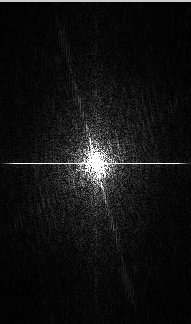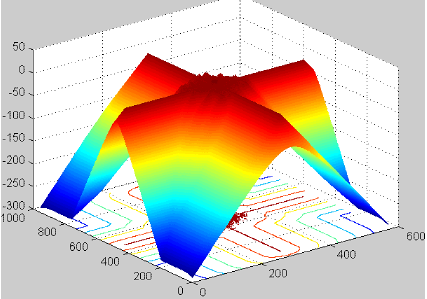由上图可以看出这张图片的中低成份占的比较多，而高频成份比较少。因为白色的像素都集中在中点和离中心的附近。对于频谱图，由白色代表某一频率点有响应，也就是原图含有该频率的成份。可以举个例子，假如我对一张图片加入椒盐噪声，这个椒盐噪声属于全频谱的。可以得到下图示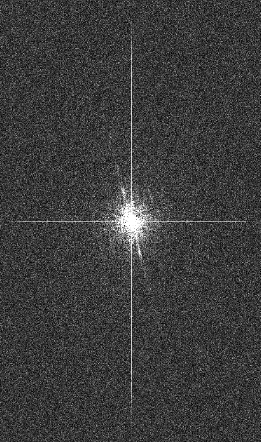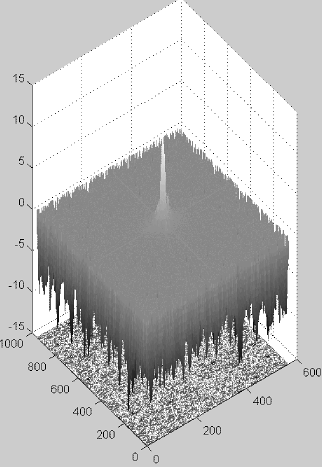很明显图像被污染了，频谱图出现了很多白色点，就代表这张图片在低频成份很多的情况下，又额外的增加了高频中频低频成份，如上第三图就出现了凹凸不平山峰一样。

解释完频率后来看带通滤波器和带阻滤波器。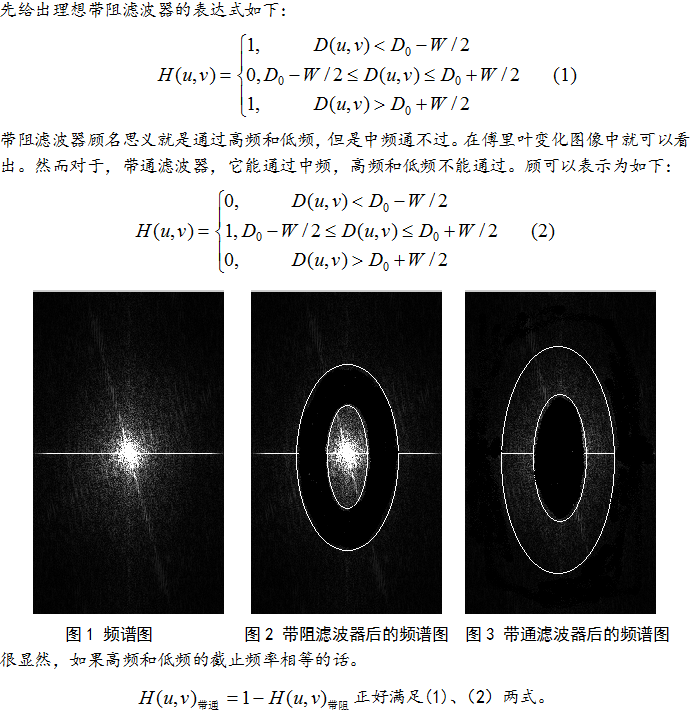再分别给出它们傅里叶反变换图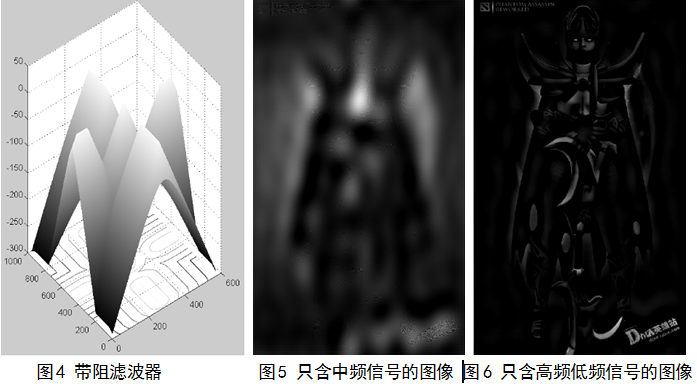图4为带阻滤波器的三维视图，图5只含中频信号的图像，图6只含高频和低频信号。读者可以自己举例子来进行高频和低频的分析，一样的原理。

只给出带阻滤波器的相关代码

I=imread('C:\Users\hlx\Desktop\1.jpg'); %读入原图像文件

I2=rgb2gray(I);

I1=double(I2);

fftI=fft2(I1); % 二维离散傅立叶变换

sfftI=fftshift(fftI); % 直流分量移到频谱中心

[N1,N2]=size(sfftI);

n=2;

d0=10;

d1=200;

n1=floor(N1/2);

n2=floor(N2/2);

for i=1:N1

for j=1:N2

d=sqrt((i-n1)^2+(j-n2)^2);

if d<=d0 || d>=d1

h=0;

else

h=1;

end

result(i,j)=h*sfftI(i,j);

end

end

sfftI=result;

RR=real(sfftI); % 取傅立叶变换的实部

II=imag(sfftI); % 取傅立叶变换的虚部

A=sqrt(RR.^2+II.^2); % 计算频谱幅值

A=(A-min(min(A)))/(max(max(A))-min(min(A)))*255; %归一化

imshow(A);


展开全文• 2、用带阻滤波器去掉频率域噪声 效果： 原图像 和 加噪声后的图像（多了很多波纹）   频率域图像 带阻滤波器   最后的图像 上代码： clear; close all; clc; I=imread('m.tif'); I=im2double(I);...
代码主要实现了两点功能

1、增加频率域噪声

2、用带阻滤波器去掉频率域噪声

效果：

原图像   和    加噪声后的图像（多了很多波纹）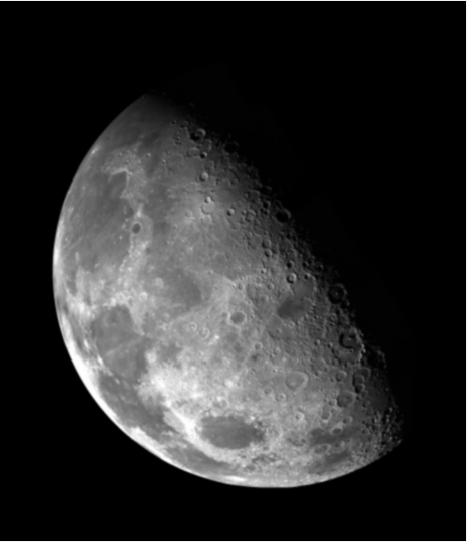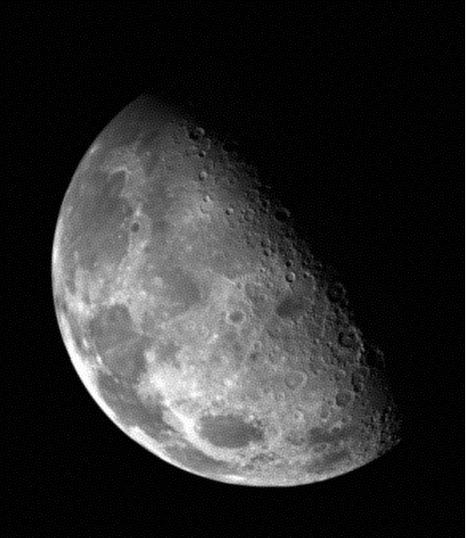频率域图像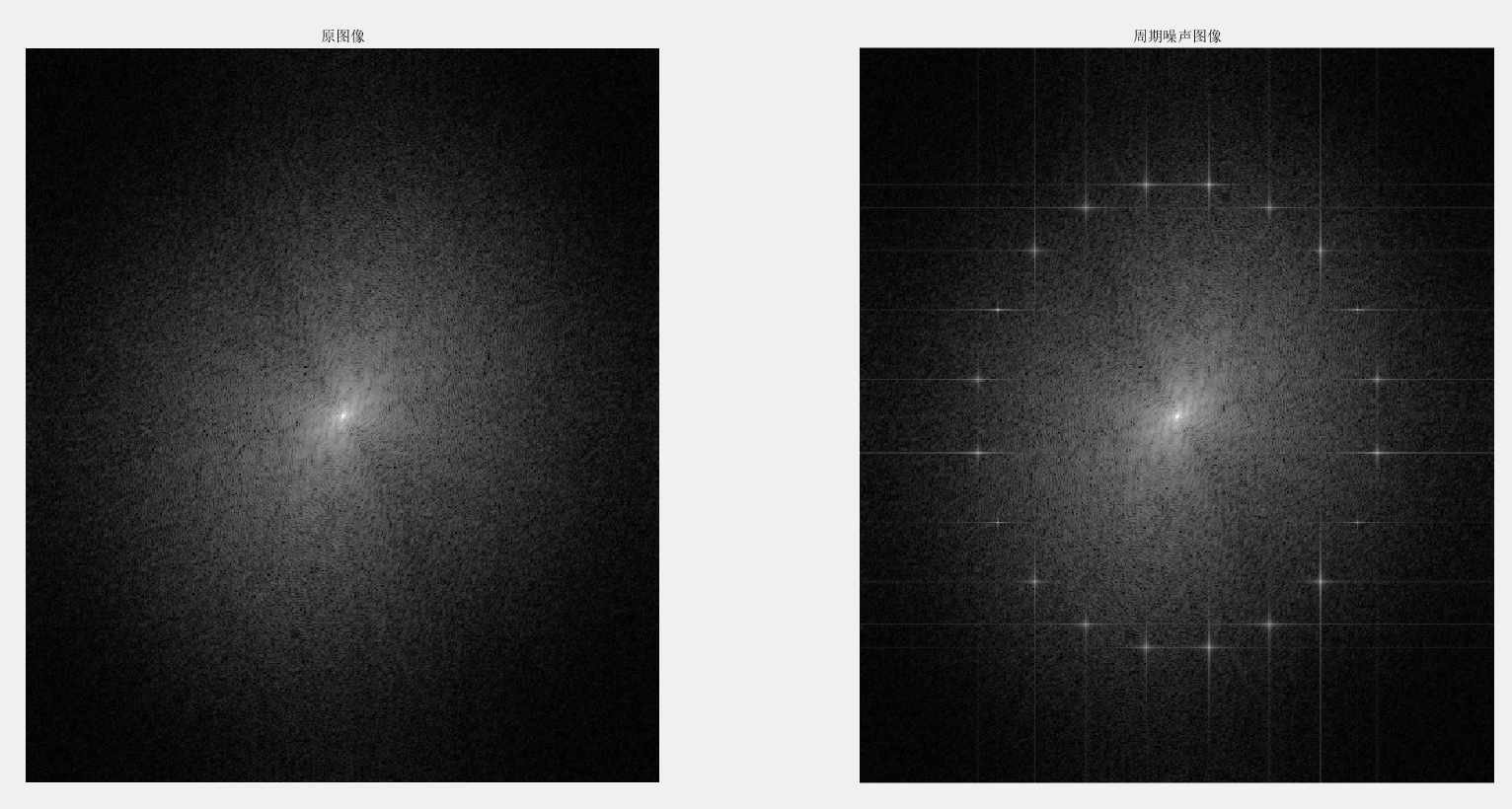带阻滤波器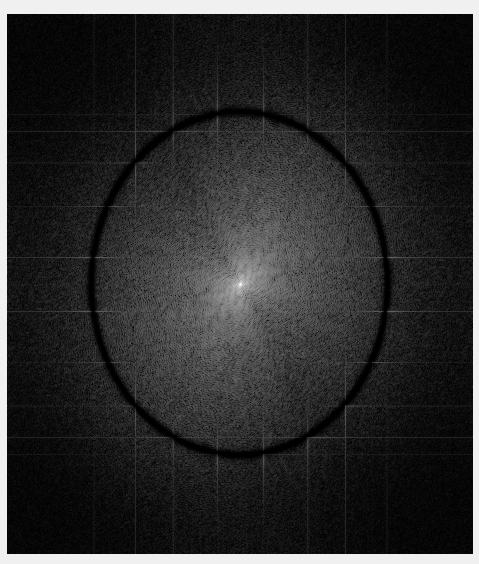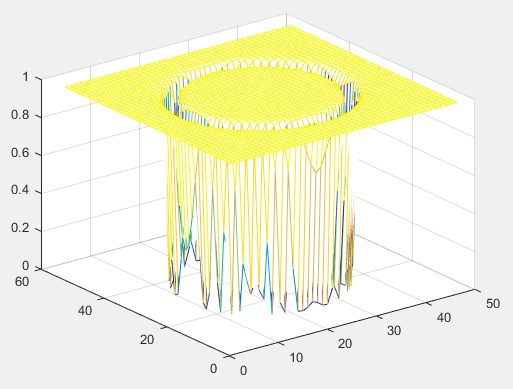最后的图像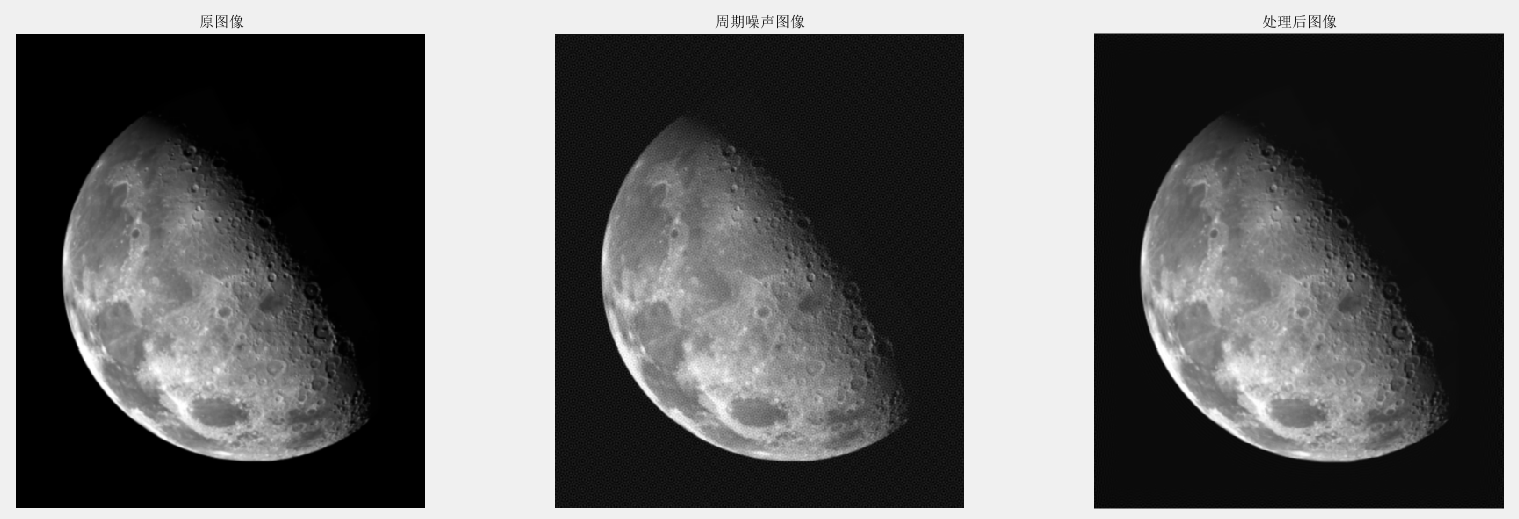上代码：

clear;
close all;
clc;

I=im2double(I);
[m,n]=size(I);

r = 0:m-1;%Row coordinates.
c = 0:n-1;%Column coordinates.
[C, R] = meshgrid(c, r);
A=0.01;
%半径2
r=2;
%划区域10个
nn=10;
f = zeros(m, n);
for i=1:nn
temp=(i-0.5)*pi/nn;
u0=r*sin(temp);
v0=r*cos(temp);
f = f+A*sin(u0*R + v0*C);
end
J=I+f;
figure;
imshow(J);

%傅里叶变换
IF=fftshift(fft2(I));
JF=fftshift(fft2(J));
%对数变换将高频细节扩展出来
IF=log(1+abs(IF));
JF=log(1+abs(JF));
figure;
subplot(1,2,1), imshow(IF,[]), title('原图像');
subplot(1,2,2), imshow(JF,[]), title('周期噪声图像');

%% %%%%%%%%%%%%%%%%%%%%%%%%%%
%处理J
k=fft2(J);
g=fftshift(k);
imshow(log(abs(g)+1),[]);

[N1,N2]=size(g);

W=10;
n=2;
d0=148;
n1=round(N1/2);
n2=round(N2/2);
Homo = zeros(N1, N2);
for i=1:N1
for j=1:N2
d=sqrt(((i-n1)*N2/N1)^2+((j-n2))^2);
Home(i,j)=1/(1+((d*W)/(d^2-d0^2))^(2*n));
end
end
figure;
mesh(abs(Home(1:10:N1,1:10:N2)));
figure;
imshow(Home,[]);

%y=g.*Home;
y=g.*Home;
figure;
imshow(log(abs(y)+1),[]);

y=ifftshift(y);
E1=ifft2(y);
E2=real(E1);

subplot(1,3,1), imshow(I,[]), title('原图像');
subplot(1,3,2), imshow(J,[]), title('周期噪声图像');
subplot(1,3,3), imshow(E2,[]), title('处理后图像');

问题：

为什么加噪声以后频率域会出现十字形状，为什么对周围造成了影响？
展开全文数字图像处理
• ## matlab设计模拟带阻滤波器

千次阅读 热门讨论 2018-10-24 22:13:11
简单记录下在matlab上如何设计出模拟的带阻滤波器，包括：巴特沃斯滤波器、切比雪夫I型滤波器、切比雪夫II型滤波器、椭圆型滤波器。 %设计带阻滤波器 %巴特沃斯、切比雪夫I型、切比雪夫II型、椭圆型滤波器 clear ...
• 低通：低频通过 高通：高频通过 带通：一定频率范围通过 带阻：阻止一定频率范围的信号  带通滤波器工作原理：  一个理想的带通滤波器应该有一个完全平坦的... 带阻滤波器工作原理：  能通过大多数频率分量...
• 1.高通滤波器  首先，对一副图像进行如下二维傅里叶变换。 我们将u=0和v=0上式，我们可以得到如下式子。 根据上式，可以到F(0,0)的值是非常大的。这里，我们将 F(0,0)称为直流分量，直流...
• 低通滤波器容许低频信号通过, 但减弱(或减少)频率高於截止频率的信号的通过。高通滤波器容许高频信号通过, ...带阻滤波器减弱(或减少)一定频率范围信号, 但容许频率低于於下限截止频率和高于上限截止频率的信号的通过。
• 带通滤波器(BPF)的特征是通带内输出信号幅度与频率无关，而当f或f>fp2时输出信号很快衰减。 二阶带通滤波器；...在低通、带阻滤波器电路中，属于DC 到AC 的直流放大电路，一般要求电路工作在双电源状态。带通滤波器
•matlab 高斯带阻滤波器
• 先给出一张图像，来说明带阻滤波器和带通滤波器的关系。   由上图可以看出这张图片的中低成份占的比较多，而高频成份比较少。因为白色的像素都集中在中点和离中心的附近。对于频谱图，由白色代表某一频率点...
• 1、低通：（Low-pass filter）是容许低于截止频率的信号通过，但高于截止频率的信号不能通过的电子...3、带通：是指能通过某一频率范围内的频率分量、但将其他范围的频率分量衰减到极低水平的滤波器，与带阻滤波器的...
• ## 滤波器中截止频率的理解

万次阅读 多人点赞 2016-12-06 23:49:55
frequency）是指一个系统的输出信号能量开始大幅下降（在带阻滤波器中为大幅上升）的边界频率。 概述 电子滤波器等信号传输通道中的诸如低通、高通、带通、带阻等频带特性都应用了截止频率的概念。截止...
• ## 频率域滤波器

千次阅读 2016-04-21 16:27:29
从滤波器处理效果的尖锐程度，可以将他们分为三种类型：理想滤波器、巴特沃斯滤波器、高斯滤波器。他们的尖锐程度也是依次递减。 再从滤波的通过范围看，这三种滤波器都有低通、高通、带通、带...巴特沃斯带阻滤波器
• 1)实现滤波器所需要的存储空间， 2) 需要计算的数目， 3)滤波器能滤掉的数量， 实际上,越多的抽头意味着有更多的阻带衰减， 更少的波纹，更窄的滤波等等。4) 乘累加 (MAC) - 在FIR方面考虑,MAC是指把延时的数据采样...
• 如何弄懂低通、高通、带通、带阻、状态可调滤波器。【低通滤波器】-（二阶压控低通滤波器）阶压控低通滤波器电路如图所示，由R1、C1 及R2、C2 分别构成两个一阶低通滤波器，但C1 接输出端，引入电压正反馈，形成压控...低通滤波器
• 利用模拟低通滤波器设计带通、带阻、高通模拟滤波器 参考：《数字信号处理 第四版》高西全、丁玉美 最近学到第六章了，利用模拟低通滤波器设计不同类型的模拟滤波器，我们重点在设计巴特沃斯滤波器。 巴特沃斯滤波器...带阻滤波器 低通滤波器 带通滤波器 高通滤波器
• ## LC滤波器原理

万次阅读 2016-03-05 09:28:54
LC滤波器原理 LC滤波器也称为无源滤波器，是传统的谐波补偿装置。...LC滤波器按照功能分为LC低通滤波器、LC带通滤波器、高通滤波器、LC全通滤波器、LC带阻滤波器；   按调谐又分为单调谐滤波器、双调谐滤波器滤波电路
...